• TF之NN：基于Tensorflow利用神经网络算法对数据集(用一次函数随机生成100个数)训练预测斜率、截距(逼近已知一次函数) 目录 输出结果 代码设计 输出结果 代码设计 import os os.environ['TF_CPP_...
TF之NN：基于Tensorflow利用神经网络算法对数据集(用一次函数随机生成100个数)训练预测斜率、截距(逼近已知一次函数)

目录
输出结果
代码设计

输出结果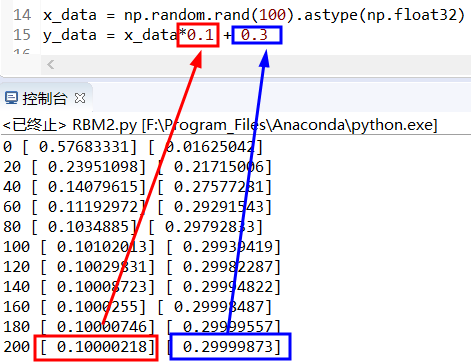代码设计
import os
os.environ['TF_CPP_MIN_LOG_LEVEL'] = '2'
import tensorflow as tf
import numpy as np

x_data = np.random.rand(100).astype(np.float32)
y_data = x_data*0.1 + 0.3

Weights = tf.Variable(tf.random_uniform(, -1.0, 1.0))
biases = tf.Variable(tf.zeros())

y = Weights*x_data + biases

loss = tf.reduce_mean(tf.square(y-y_data))
train = optimizer.minimize(loss)

#init = tf.initialize_all_variables()
init = tf.global_variables_initializer()

### create tensorflow structure end ###

sess = tf.Session()
sess.run(init)

for step in range(201):
sess.run(train)
if step % 10 == 0:
print(step, sess.run(Weights), sess.run(biases))
相关文章TF：Tensorflow之一次函数应用，随机生成100个数，利用Tensorflow训练使其逼近已知一次函数的斜率和截距


展开全文• tensorflow单变量线性回归（一次函数拟合） 本文章为本人自己写的jupyter笔记，图片显示有一点问题（下面的每个图片都变成同一张图片），所以仅供预览。 需要下载ipynb文件的朋友可以关注我并找到我上传的资源。 ....
Tensorflow单变量线性回归（一次函数拟合）
本博客为本人自己整理的jupyter笔记，现在以图片形式上传，所以仅供预览。
需要下载ipynb文件的朋友可以关注我并找到我上传的资源。 https://download.csdn.net/download/weixin_43907422/11131647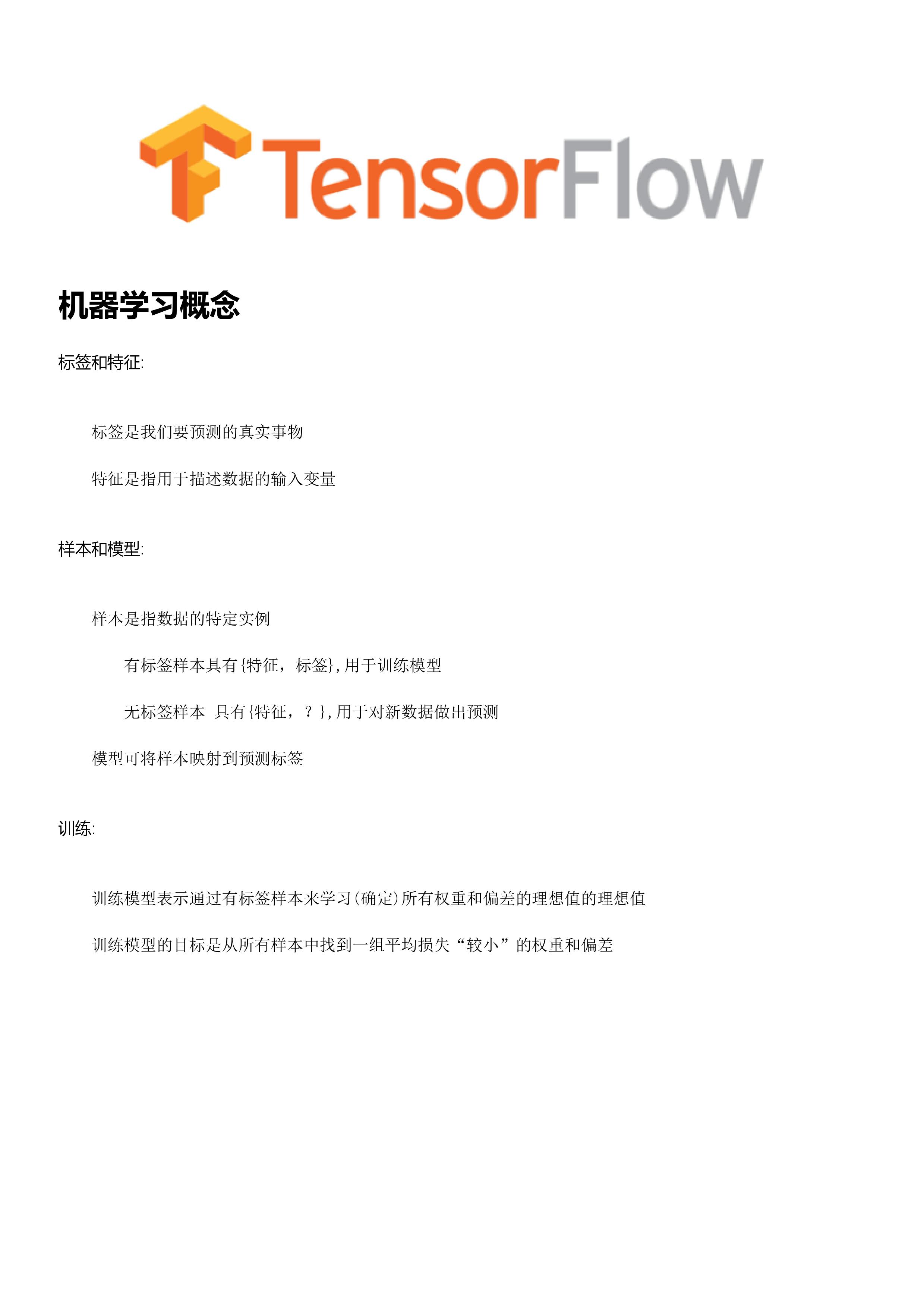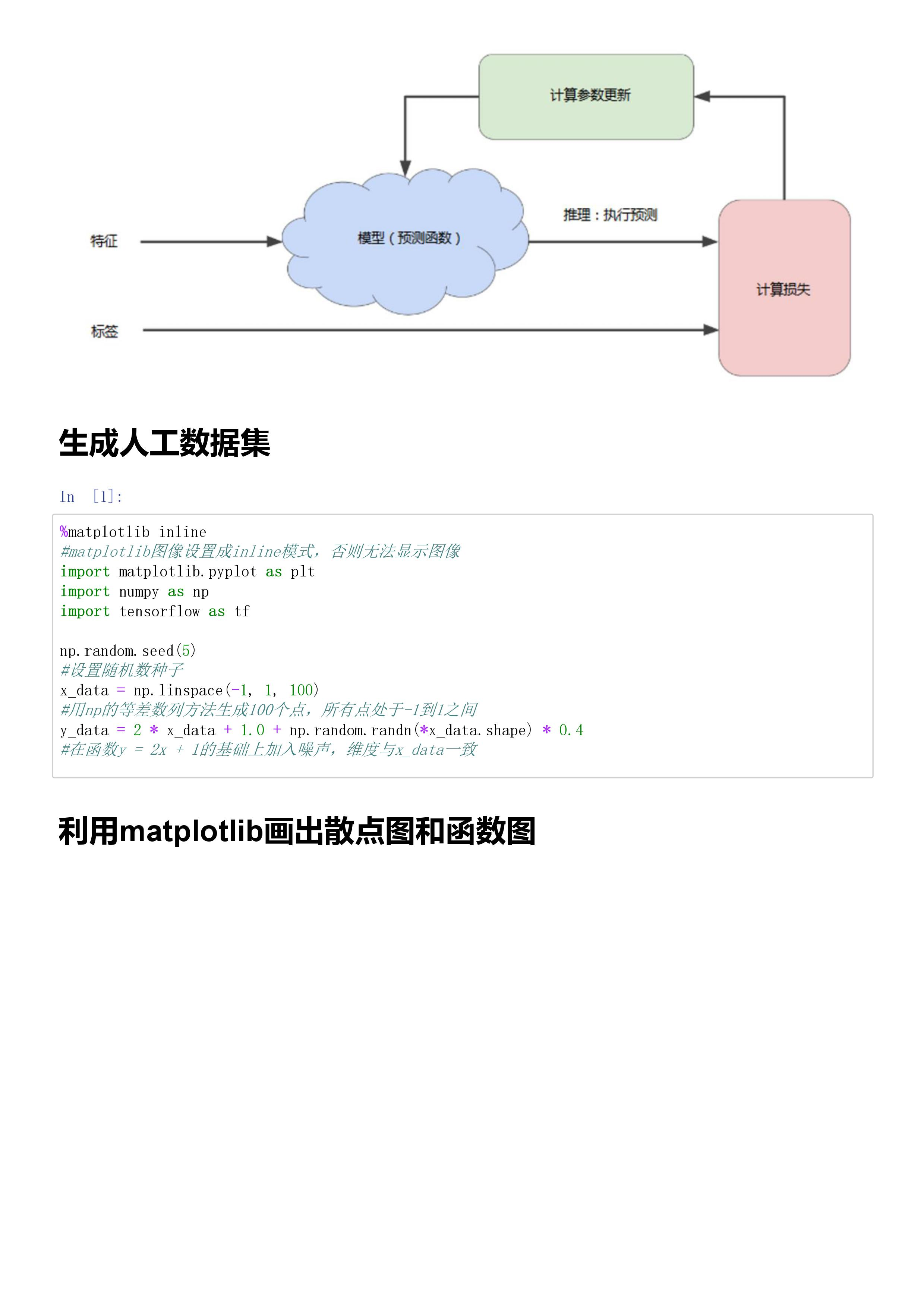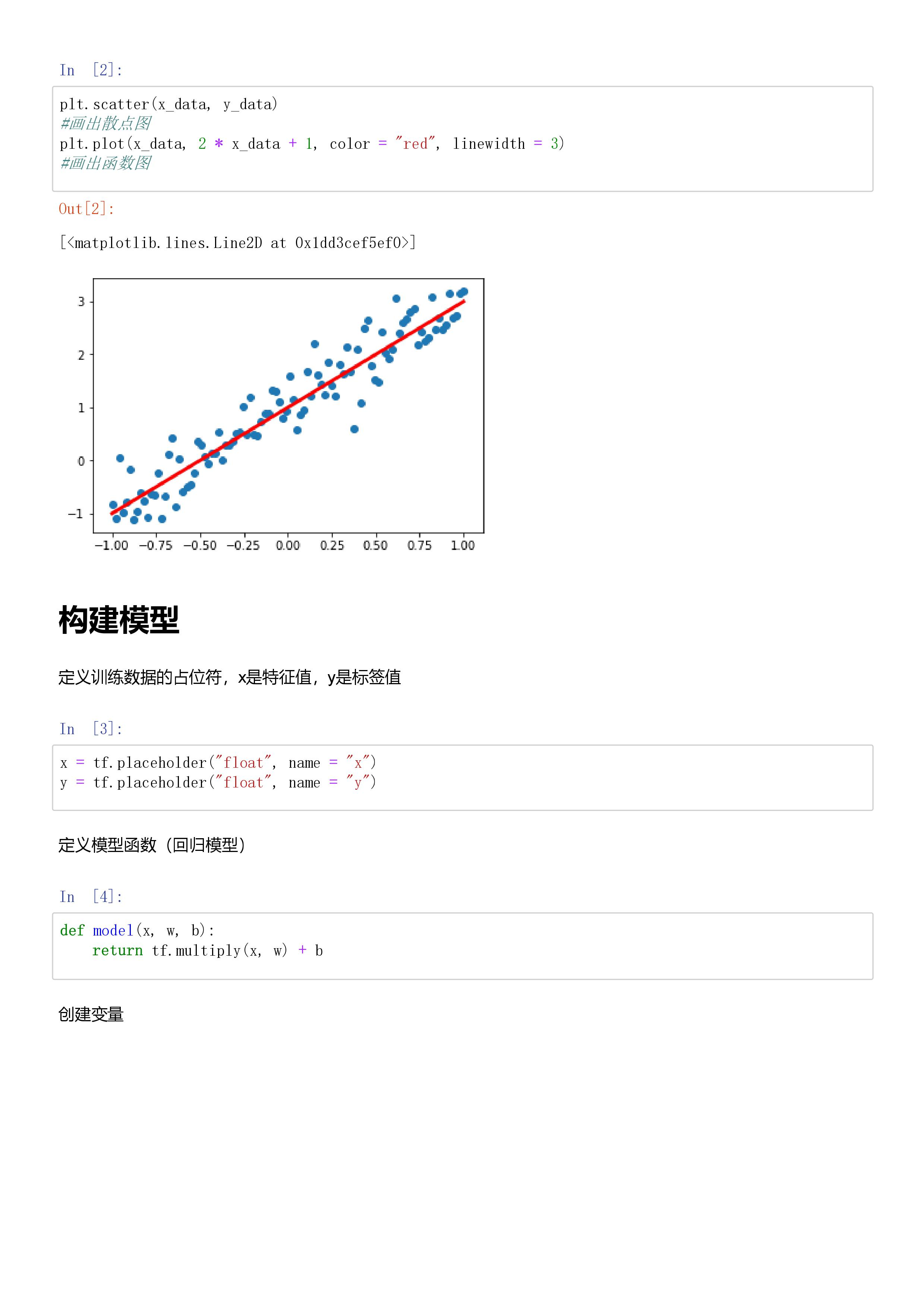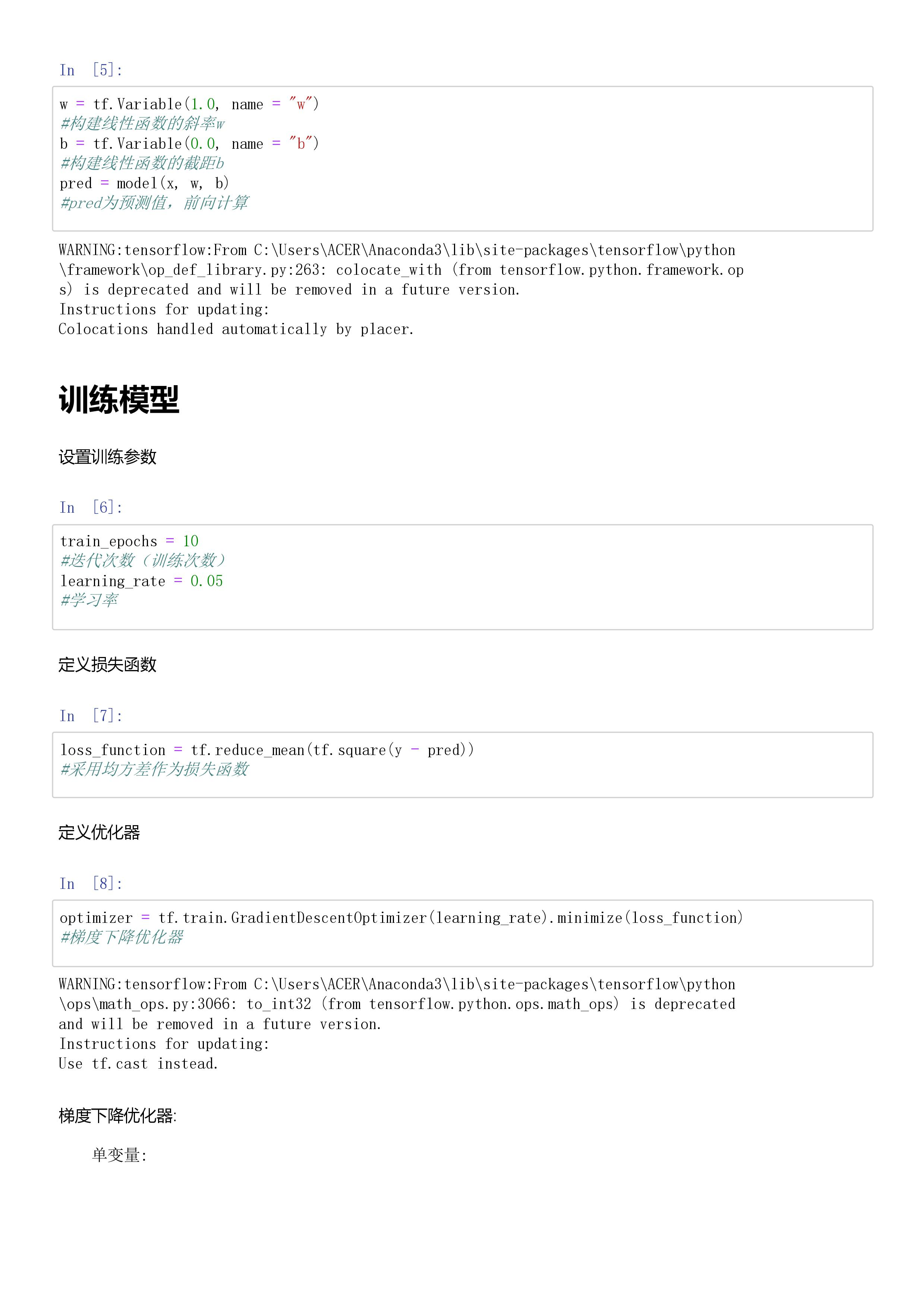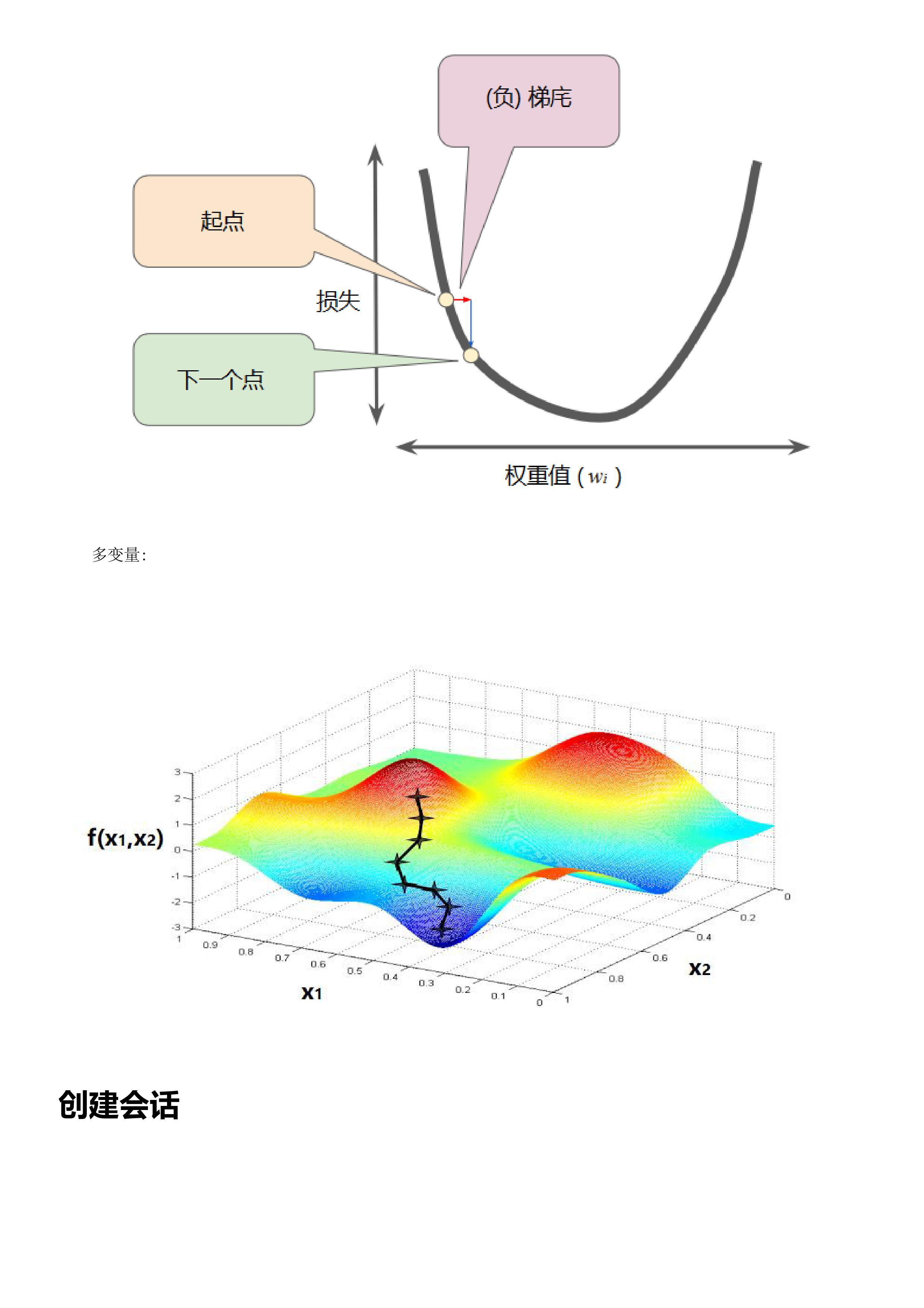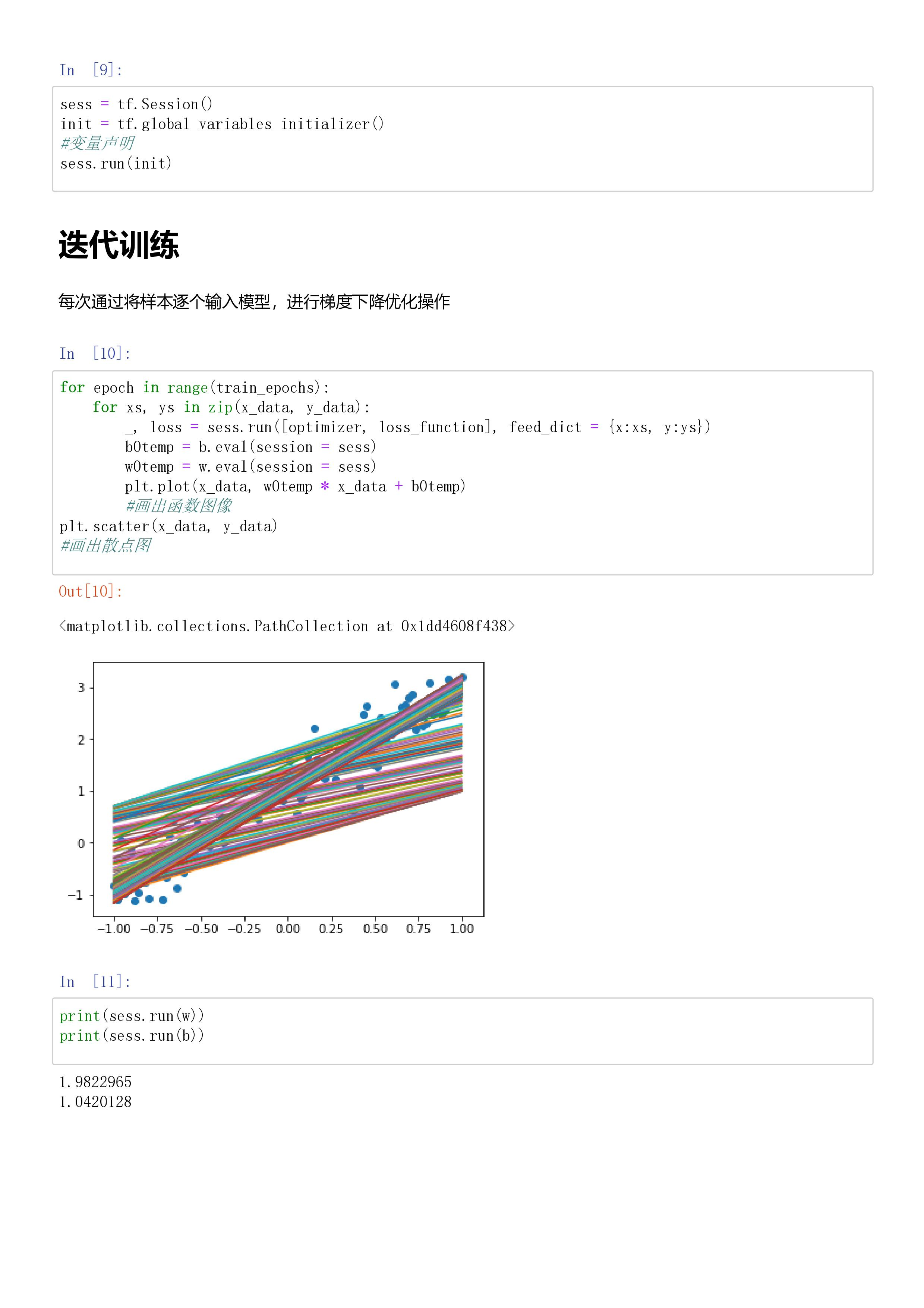2019年4月26日
展开全文tensorflow 线性回归
• 每隔一定时间运行一次函数/任务 例子中每10秒执行一次… def func_task(): print('执行任务中...') def func_timer(): func_task() global timer # 定义全局变量 # 定时器构造函数主要有2个参数，第一个参数为...
每隔一定时间运行一次函数/任务 例子中每10秒执行一次…
def func_task():
print('执行任务中...')

def func_timer():

global timer  # 定义全局变量
# 定时器构造函数主要有2个参数，第一个参数为时间，第二个参数为函数名
timer = threading.Timer(10, func_timer)   # 10秒调用一次函数

print("线程名称={},\n正在执行的线程列表:{},\n正在执行的线程数量={},\n当前激活线程={}\n".format(
)

timer.start()    #启用定时器

timer.start()
print('定时器启动成功-----')

展开全文• 使用scipy拟合一元二次函数。 参考： HYRY Studio-《用Python做科学计算》 代码： import numpy as np from scipy.optimize import leastsq import pylab as pl def func(x,p): """ 数组拟合函数 """ A,k,...
背景：

使用scipy拟合一元二次函数。

参考：

HYRY Studio-《用Python做科学计算》

代码： import numpy as np
from scipy.optimize import leastsq
import pylab as pl
def func(x,p):
"""
数组拟合函数
"""
A,k,theta = p
return A*(x-k)**2+theta
def residuals(p,y,x):
"""
残差
"""
return y-func(x,p)
x = np.linspace(0,2,100)
A,k,theta = 10.,1,2. #真实数据参数
y0 = func(x,[A,k,theta]) #真实数据
y1 = y0 + 2 * np.random.randn(len(x)) #加入噪声序列
p0 = [7.,0.2,1.]
plsq = leastsq(residuals,p0,args = (y1,x))
print("真实参数:",[A,k,theta])
print("拟合参数:",plsq) #试验数据拟合后的参数
pl.plot(x,y0,label = "real")
pl.plot(x,y1,label = "real+noise")
pl.plot(x,func(x,plsq),label = "fitting")
pl.legend()
pl.show() 结果： （貌似这里的求解方法用了智能算法，因为每次的结果都有细小差异。具体资料没见到，以后有精力再找） 真实参数: [10.0, 1, 2.0] 拟合参数: [ 11.06873474   0.99709893   1.49107462]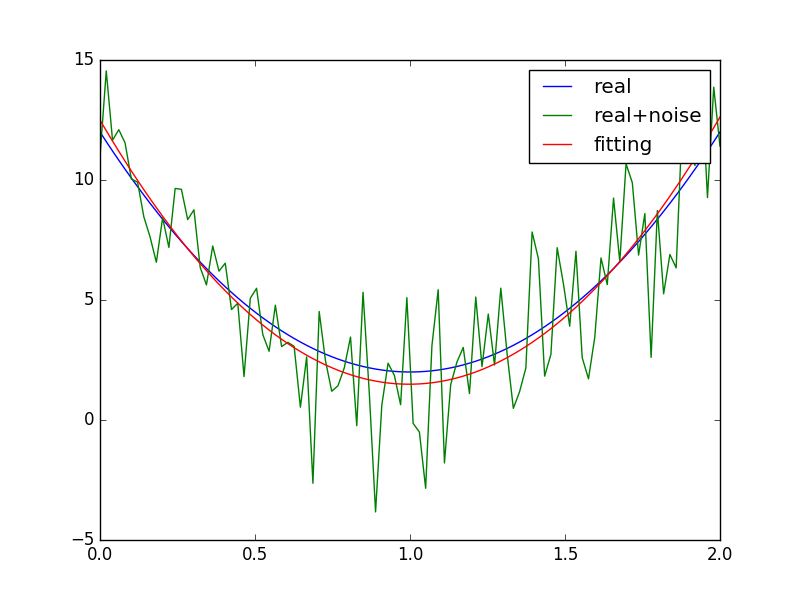展开全文python numpy scipy
• #代码如下： import matplotlib.pyplot as plt import numpy as np x=np.linspace(-1,0,5) y=-2*x plt.plot(x,y) plt.show()
• 这个方法中传入了两个参数，一个是函数引用，注意，是函数引用，所以后面不能加（），第二个参数是一个时间，它的意思是，每隔一定时间执行一次传入的函数。 比如，这里写一个显示当前时间的函数： var time=new...javascript
• function f(){ var cnt=0; return function(){ return ++cnt;} } var fa=f();//将函数f的的返回值给变量fn ...函数的返回值是函数（对象的引用），这里将其赋值给变量fn。在调用fn时，其输出结果每次都...
• ## MATLAB画函数图像

万次阅读 多人点赞 2019-04-19 15:56:34
1 画图基础 （1）一元一次函数 x=0:0.1:1; y=x; plot(x,y); %图像见下图1 图1 ...
• 第一张图是当模型为一元一次函数时的情况，以及其loss函数（二元二次函数）的图像是如何由函数的子项形成的，以及二元二次函数梯度的不同对学习率的影响。一般来说采用全量梯度下降时函数图像最陡，批量梯度下降次之...机器学习 回归模型 最小二乘法 理论分析
• 二次函数是中学时代必学的一类基本初等函数，是在学习了一次函数的基础上再进行研究的，在学习时要学会掌握画函数图像的方法，之前都是借助直尺来画函数图像的，现在可以借助专业的绘图工具来完成，下面就一起来学习...
• ## 次模函数

千次阅读 2017-01-31 21:52:22
前言本篇小记要介绍个解析函数上面的概念——函数（Submodular Function）。 函数也称作“子模函数”或“亚模函数”，具有模型（Submodularity），也称“子模性”或“亚模性”，它是经济学上的概念——...计算机数学 submodular
• 函数体里覆写当前函数，那么可以创建一个一次性的函数，重新赋值之前的代码相当于只运行了一次，适用于运行一些只需要执行一次的初始化代码 var sca = function() { console.log('msg') sca = function() { ...js
• 一个只执行一次函数(最优解在下面) function once(func) { var ran, result; if (!isFunction(func)) { throw new TypeError(funcErrorText); } return function() { if (r...
• #include #include int main() { float a=0; float b=3;  float c=2; float x1,x2; float m=b*b-4*a*c;... printf("该函数不是一元二次函数\n"); printf("x = %lf\n",x1); } else {
• 一次函数、二次函数、反比例函数、指数函数、对数函数、幂函数的性质与图像知识点的总结； 今天高中数学必修一函数性质图像的知识点就分享到这里，有更多高中数学学习方法以及解题技巧需要的可以私聊...高中数学
• ## Python绘制函数图像

千次阅读 多人点赞 2020-06-03 21:44:09
一元一次函数 一元二次函数 指数函数 正余弦函数 二元一次函数 二元二次函数 导入相关包 from mpl_toolkits.mplot3d import Axes3D import numpy as np from matplotlib import pyplot as plt plt.rcParams...python 可视化
• ## js原生函数节流

万次阅读 多人点赞 2020-04-29 17:27:11
函数节流就是一定时间内只触发一次函数。原理是通过判断是否到达一定时间来触发函数。 // 时间戳方案 function throttle(fn,wait){ var pre = Date.now(); return function(){ var context = this; var args =...
• 方法： 如果定义域不关于原点对称，则该函数没有...偶函数：若对于定义域内的任意个x，都有f(-x)=f(x)，那么f(x)称为偶函数。 奇函数：若对于定义域内的任意个x，都有f(-x)=-f(x)，那么f(x)称为奇函数。 ...
• setTimeout (function(){    var i=0;   var handler = setInterval (function(){ ...可以把 var handler 变量 定义为全局变量 ， 这样可以在其他函数中 清除 setInterval 调用的函数
• 高中数学的学习有几个方面的知识点组成：集合、函数（包含一次函数，二次函数，指数函数，对数函数，三角函数）、几何（直线方程，圆的方程）、概率、向量等等。 其中学生们学习最有难度的模块就属于函数和几何了，...高中数学
• 为什么个c程序只能有个main函数？如果个程序有多个功能，那么不能有多个main函数么？
• 今天复习遇到了函数主体使用多scanf函数的情况。但是在实际运行中发现只有第个scanf正常赋值了，之后的scanf函数均未赋值，被赋值为回车。 代码： #include #include double cal(double a1, double b1...
• //设计个递归函数，求x的n幂： //主函数通过键盘输入x和n的值，并输出x^n的值（假设x为实数，n为正整数） double fun(double x,int n){ if(n==1) return x; return x*fun(x,n-1); } int main() { double x;...
• - 一元一次函数 - 一元二次函数 - 指数函数 - 自然对数函数 - 正弦函数 一元一次函数 效果图 代码 import matplotlib.pyplot as plt import numpy as np x = np.arange(0, 10, 0.1) y = x * 2 plt.title(
• 看完文章，长点见识。...人为选定采用二次函数的方法拟合。 收集的数据如表： 那么就不难列出如下个方程： 价格=系数阵*数据阵 代码： c=data_new\price 其中系数阵就是不同下标的C，其中数据阵就是【V^2...matlab
• 一次调用，两次返回-------fork函数学习总结  以前一直迷惑，什么叫一次调用，两次返回。通过上网搜索，终于知其原由。现将自己的理解记录于此。  准备知识：  内存中的进程包括三个部分：可执行文件（即程序...
• 1. 定义标记变量 data() { ... firstPlayFlag: true, // 第一次播放标记 } } 2.应用 if（this.firstPlayFlag） { // 执行函数 this.firstPlayFlag = false } else { } ...
• 函数中定义静态变量，虽多次调用函数，但只定义一次！！！！  少废话，先上代码 #include using namespace std; class A { public: A(int a1) :a(a1) {} int a; }; //多次调用，只定义一次，返回同一个 A ...
• 通过一道面试题详细解读js函数内返回内部函数的问题。...